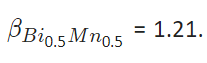# Conversion of the Bohr Magneton Number

It happens quite often that a phase was modelled using different chemical formulas in different thermodynamic assessments. In this situation, if the contribution from magnetic ordering is involved, the value used for the Bohr magneton number ought to be different to have the same magnetic contribution to the molar Gibbs energy of the phase. Unfortunately, many mistakes can be found in publications regarding the conversion of this important magnetic parameter. In this blog, I present the proper equations for the conversion.

The relationship between the Bohr magneton numbers involved can be derived as follows:

Supposing the following chemical formula is used in one thermodynamic description with a Bohr magneton number as β,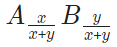now in a new thermodynamic modelling, a different chemical formula is used with a new Bohr magneton number as β’ :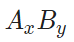In order to have the same molar magnetic Gibbs energy, it holds:

Eq. 1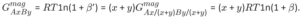Eq. 2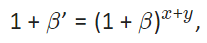Eq. 3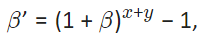Eq. 4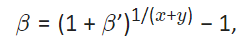For example supposing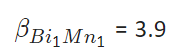and using Eq 4, we get